# Multiplying Fractions By Cancelling Common Factors

Example 1: Multiply.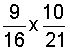Solution: We will multiply these two fractions, and then simplify the result.

Step 1: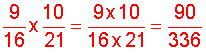Step 2: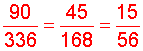There are two ways to solve this problem:

1. We can reduce the result to lowest terms as we did above. This can get tedious, especially given the large numbers in the problem.
2. We  can cancel (divide out) common factors before we multiply.

Let's look at the second method: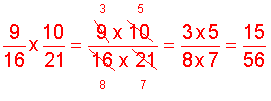We divided 3 into 9 and 21, and we divided 2 into 10 and 16. The product is a fraction that is reduced to lowest terms. Let's look at more examples of multiplying fractions with cancelling common factors.

Example 2: Multiply by dividing out common factors.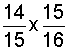Analysis: Divide 15 into 15. Divide 2 into 14 and 16.

Solution: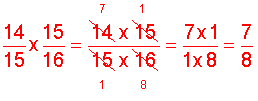The following is the procedure for multiplying fractions with cancelling of factors.

Procedure: To multiply fractions by cancelling common factors, divide out factors that are common to both a numerator and a denominator. The factor being divided out can appear in any numerator and any denominator.

Let's look at some examples using this procedure.

Example 3: Multiply by dividing out common factors.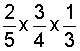Analysis: Divide 3 into 3. Divide 2 into 4 and 2.

Solution: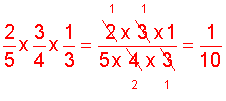As you can see, this method is also useful for multiplying more than two fractions.

Example 4: Multiply by dividing out common factors.Analysis: We are multiplying a whole number by a fraction. First, convert the whole number to an improper fraction. Next, divide 8 into 8 and 144.

Solution: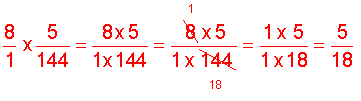Example 5: Multiply by dividing out common factors.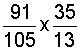Analysis: Divide 13 into 13 and 91. Divide 35 into 35 and 105.

Solution: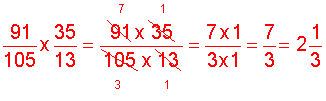Example 6: Multiply by dividing out common factors.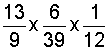Analysis: In this problem, we will cancel and then cancel again.

Solution:Note: First, we divided 13 into 13 and 39; and divided 3 into 3 and 6. Next, we divided 2 into 2 and 2 into 12.

In example 6, there is more than one way to cancel out the factors. The order we chose is listed above in the note section. However, it is possible to cancel out factors in a different order. You should still arrive at the same answer, regardless of the order you choose.

Summary: To multiply fractions by cancelling common factors, divide out factors that are common to both a numerator and a denominator. The factor being divided out can appear in any numerator and any denominator.

### Exercises

Directions: In each exercise below, multiply the fractions by dividing out common factors. Be sure to simplify your result, if necessary. Click once in an ANSWER BOX and type in your answer; then click ENTER. After you click ENTER, a message will appear in the RESULTS BOX to indicate whether your answer is correct or incorrect. To start over, click CLEAR.

Note: To write the fraction three-fourths, enter 3/4 into the form. To write the mixed number four and two-thirds, enter 4, a space, and then 2/3 into the form.

 1.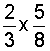ANSWER BOX:   RESULTS BOX:
 2.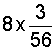ANSWER BOX:   RESULTS BOX:
 3.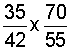ANSWER BOX:   RESULTS BOX:
 4.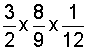ANSWER BOX:   RESULTS BOX:
 5.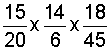ANSWER BOX:     RESULTS BOX:

 Lessons on Multiplying and Dividing Fractions and Mixed Numbers 1. Multiplying Fractions 2. Multiplying Fractions by Cancelling Common Factors 3. Multiplying Mixed Numbers 4. Reciprocals 5. Dividing Fractions 6. Dividing Mixed Numbers 6. Solving Word Problems 7. Practice Exercises 8. Challenge Exercises 9. Solutions